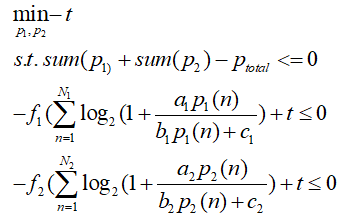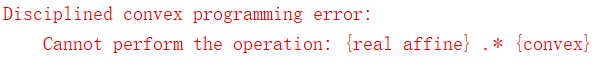# How can I transform the constraint condition ini order that the condition is cvx_solvable

Recently I’m working on the simulation about comunication, and I meet a optimization problem which makes me under too much presure.where t ,p1(N1), p2(N2) are the variables , and f1, f2 are constants. a1, a2, b1, b2, c1, c2 are the real matrix which is known to us.
I developed the code below in cvx

but the output of the matlab is thatHow can I avoid the bug and express the constraint condition correctly?
What’s more, I found that the constraint condition is not convex when a1(n)<b1(n), a2(n)<b2(n), I’m under too much presure because of the question. Would you please tell me how to transform the constraint condition into standard form or using some inequality relations on them?
Would you please tell me how to deal with the problems if you kown something about them? I appreciate your help very much!

I think you can apply the reformulation in Can CVX solve this kind of function {x-log(1+0.01*x/(x+1))} to each summation term in the constraints.

In the future, please copy and paste code into your post, using Preformatted text icon, rather than posting an image of your code.

Thanks so much for your professional answer!!! The cvx constraint condition question is solved by using the method that

[quote=“Mark_L_Stone, post:2, topic:9149, full:true”]
I think you can apply the reformulation in Can CVX solve this kind of function {x-log(1+0.01*x/(x+1))} to each summation term in the constraints.

In the future, please copy and paste code into your post, using Preformatted text icon, rather than posting an image of your code.
[/quote]. Thank you very very much!!! I will not use the picture to represent my question any more. I’m so so sorry about that.
In my simulation, I meet another question which troubled me.
I use the cvx 2.2 and mosek 9.1.9 to solve the question
cvx_begin
variable t
variable p1(N1,1)
variable p2(N2,1)
minimize(-t)
subject to
(-1)*t<=0;
for n1=1:N1
(-1)*p1(n1,1)<=0;
end
for n2=1:N2
(-1)*p2(n2,1)<=0;
end
sum(p1)+sum(p2)-p_total<=0;
for i1=1:NO1
value1=0;
for ii1=1:N1
value1=value1+log(1+B1_Cell{i1,5}(ii1,1)-B1_Cell{i1,5}(ii1,1)*B1_Cell{i1,6}(ii1,1)*inv_pos(p1(ii1,1)+B1_Cell{i1,6}(ii1,1)))/log(2);
end
(-1)*value1+t<=0;
end

``````        for i2=1:NO2
value2=0;
for ii2=1:N2
value2=value2+log(1+B2_Cell{i2,5}(ii2,1)-B2_Cell{i2,5}(ii2,1)*B2_Cell{i2,6}(ii2,1)*inv_pos(p2(ii2,1)+B2_Cell{i2,6}(ii2,1)))/log(2);
end
(-1)*value2+t<=0;
end
cvx_end
``````

At first few times, the matlab output is normal, however, after few times, the output of matlab becomes :
Optimizer started.
Presolve started.
Linear dependency checker started.
Linear dependency checker terminated.
Eliminator started.
Freed constraints in eliminator : 0
Eliminator terminated.
Eliminator started.
Freed constraints in eliminator : 0
Eliminator terminated.
Eliminator - tries : 2 time : 0.00
Lin. dep. - tries : 1 time : 0.00
Lin. dep. - number : 0
Presolve terminated. Time: 0.03
Problem
Name :
Objective sense : min
Type : CONIC (conic optimization problem)
Constraints : 1039
Cones : 1048
Scalar variables : 3169
Matrix variables : 0
Integer variables : 0

Optimizer - solved problem : the primal
Optimizer - Constraints : 1039
Optimizer - Cones : 1048
Optimizer - Scalar variables : 3169 conic : 3144
Optimizer - Semi-definite variables: 0 scalarized : 0
Factor - setup time : 0.00 dense det. time : 0.00
Factor - ML order time : 0.00 GP order time : 0.00
Factor - nonzeros before factor : 7605 after factor : 1.59e+04
Factor - dense dim. : 0 flops : 4.89e+05
ITE PFEAS DFEAS GFEAS PRSTATUS POBJ DOBJ MU TIME
0 1.5e+01 1.5e+15 1.2e+04 0.00e+00 1.150469465e+04 0.000000000e+00 1.0e+00 0.06
1 7.0e+00 7.3e+14 8.0e+03 -1.00e+00 1.151098091e+04 7.354833266e+00 4.8e-01 0.17
2 1.1e+00 1.1e+14 3.1e+03 -1.00e+00 1.149712701e+04 4.931274102e+00 7.4e-02 0.19
3 2.6e-01 2.7e+13 1.5e+03 -1.00e+00 1.145411878e+04 4.627260324e+00 1.8e-02 0.20
4 1.7e-01 1.8e+13 1.3e+03 -1.00e+00 1.142662690e+04 4.376172671e+00 1.2e-02 0.22
5 4.6e-02 4.8e+12 6.5e+02 -1.00e+00 1.119203505e+04 2.910704591e+00 3.2e-03 0.22
6 1.2e-02 1.2e+12 3.3e+02 -1.00e+00 1.028178796e+04 1.935104668e+00 8.2e-04 0.23
7 2.9e-03 3.0e+11 1.6e+02 -1.00e+00 6.488447257e+03 1.570595044e+00 2.0e-04 0.25
8 7.3e-04 7.6e+10 8.1e+01 -1.00e+00 -8.479353955e+03 1.120403332e+00 5.0e-05 0.27
9 1.6e-04 1.7e+10 3.8e+01 -1.00e+00 -7.992730275e+04 8.586086394e-01 1.1e-05 0.28
10 2.0e-05 2.1e+09 1.3e+01 -1.00e+00 -7.212370065e+05 7.795012563e-01 1.4e-06 0.28
11 2.5e-06 2.6e+08 4.8e+00 -1.00e+00 -5.851586243e+06 6.985123830e-01 1.7e-07 0.30
12 2.9e-07 3.0e+07 1.6e+00 -1.00e+00 -5.011191746e+07 5.715748955e-01 2.0e-08 0.31
13 3.0e-08 3.1e+06 5.2e-01 -1.00e+00 -4.899735512e+08 -2.764072256e-03 2.0e-09 0.31
14 3.9e-10 4.1e+04 5.8e-02 -1.00e+00 -3.713480280e+10 -6.837846132e-03 2.7e-11 0.33
Optimizer terminated. Time: 0.39

Interior-point solution summary
Problem status : DUAL_INFEASIBLE
Solution status : DUAL_INFEASIBLE_CER
Primal. obj: -9.9998258127e-01 nrm: 7e+01 Viol. con: 3e-09 var: 3e-11 cones: 0e+00
Optimizer summary
Optimizer - time: 0.39
Interior-point - iterations : 14 time: 0.33
Basis identification - time: 0.00
Primal - iterations : 0 time: 0.00
Dual - iterations : 0 time: 0.00
Clean primal - iterations : 0 time: 0.00
Clean dual - iterations : 0 time: 0.00
Simplex - time: 0.00
Primal simplex - iterations : 0 time: 0.00
Dual simplex - iterations : 0 time: 0.00
Mixed integer - relaxations: 0 time: 0.00

Status: Infeasible
Optimal value (cvx_optval): +Inf

the picture means that：
A nonexistent field ‘sol’ was referenced
error in cvx_mosek
error in cvxprob/solve
error in cvx_end
error in optimization method

Is my matrix’s column number to large? or because the optimization function became to large? I try to decrease matrix’s column and the value1’number ,but the problem still exits. Would you tell me how to deal the problem if you know somthing ahout it? I really appreciate your help!!!

You show output indicating the problem was determined (primal) infeasible, and then you show an error message in red.

I don’t understand “when” the error message in red occurred. is there an outer loop around your code in which the output from one “iteration” of this outer loop is used as input to the CVX problem the next time through the loop? if so, you get into trouble when the previous iteration resulted in infeasible, and therefore the “optimal” variable values from the previous iteration are `NaN`, which results in CVX errors due to “invalid” when used in a CvX expression. But I don’t see an “invalid” error message.

Using your own crude (non-safeguarded) iterative"Successive Convex Approximation" algorithm around CVX is fraught with peril. This forum has many example of such failed algorithm attempts. You may be better of using a non-convex optimization solver instead.

Perhaps this is not the reason for the error message. You will have to be clearer on exactly where and when it does or does not occur, an d what your sequence of CVX problems actually is.

## Thank you very much for your analysis of my question! There is a outer loop around my code, but each loop is independent, the input or output of the current itration has no relation to the input or output of the next loop. What’s more, I found this error information in the output of matlab Calling Mosek 9.1.9: 3515 variables, 1172 equality constraints For improved efficiency, Mosek is solving the dual problem.

MOSEK Version 9.1.9 (Build date: 2019-11-21 11:34:40)